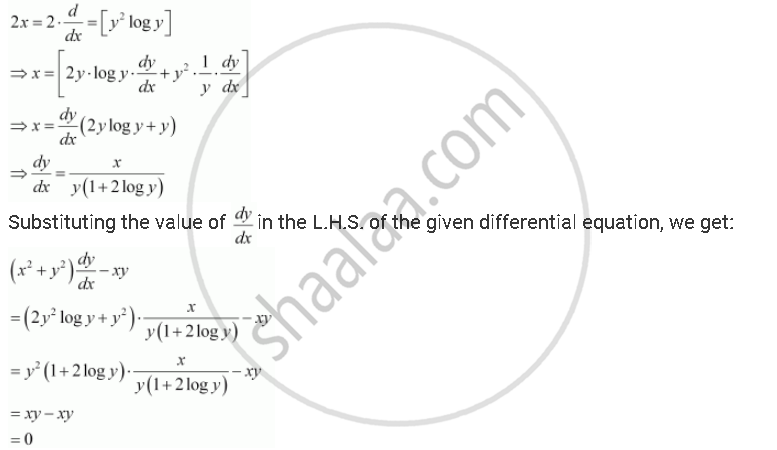Share

# For Given Below, Verify that the Given Function (Implicit Or Explicit) is a Solution of the Corresponding Differential Equation. X^2 = 2y^2 Log Y : (X^2 + Y^2) Dy/Dx - Xy = 0 - CBSE (Science) Class 12 - Mathematics

ConceptOrder and Degree of a Differential Equation

#### Question

For given below, verify that the given function (implicit or explicit) is a solution of the corresponding differential equation.

x^2 = 2y^2 log y    :  (x^2  + y^2) dy/dx - xy = 0

#### Solution

x^2 = 2y^2 log y

Differentiating both sides with respect to x, we get:Hence, the given function is a solution of the corresponding differential equation.

Is there an error in this question or solution?

#### APPEARS IN

NCERT Solution for Mathematics Textbook for Class 12 (2018 to Current)
Chapter 9: Differential Equations
Q: 2.4 | Page no. 420

#### Video TutorialsVIEW ALL 

Solution For Given Below, Verify that the Given Function (Implicit Or Explicit) is a Solution of the Corresponding Differential Equation. X^2 = 2y^2 Log Y : (X^2 + Y^2) Dy/Dx - Xy = 0 Concept: Order and Degree of a Differential Equation.
S